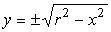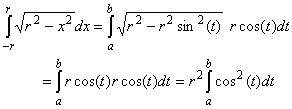#Interactive Real Analysis

Next | Previous | Glossary | Map

## 7.2. Integration Techniques

### Example 7.2.4(c): Applying the Substitution Rule

Compute the area of a circle with radius r
Of course everyone knows that the correct answer isr2, but we want to compute the answer, not just state it.
 First we need to describe the circle mathematically. We know that x2 + y2 = r2 is a circle, which we can solve for y:where the positive root gives the upper half-circle (blue) and the negative one the lower part (red).
As for the area of the circle, we clearly have:Now we need to guess a change of variables that will make this integrand easier. Since we are dealing with a circle, the trig functions sin and cos might come to mind, and especially the fact that sin(t)2 + cos(t)2 = 1, or equivalently
r2 - (r cos(t))2 = (r sin(t))2
Therefore we try the following substitution:
x = r sin(t) so that
dx/dt = r cos(t) or dx = r cos(t) dt
With that substitution the integral is transformed into a much simpler version:where we determine the new integration interval [a, b] later. Now we need to remember a few facts about trig functions, in particular the so-called "double-angle" formula:
cos2(t) = 1/2 (cos(2t) + 1)
Therefore our area is
A = 2 r2/2cos(2t) + 1 dt = r2 [cos(2t) dt +1 dt ] =
= r2 [1/2 ( sin(2b) - sin(2a) ) + (b - a)]
where we have used the substitution u = 2t in our head to evaluate the first integral.

It remains to find the values of a and b. They originate from the original substitution of x = r sin(t). Therefore x = -r must correspond to a such that r sin(a) = -r and x = r must correspond to b where r sin(b) = r:

-r = r sin(a) and r = r sin(b)
Therefore a = -/2 and b =/2. Now we can compute the final answer:
A = r2 [1/2 ( sin(2b) - sin(2a) ) + (b - a)] =
= r2 [1/2 ( sin() - sin(-) +] =
=r2
Lucky us, we got the correct answer! Note that this time our change of variables is different from our previous examples:
• usually we change an expression in x to a single variable u.
• this time we changed a single variable x to an expression in another variable t.
But as long as we correctly transform the dx term based on our substitution, anything goes.
Next | Previous | Glossary | Map